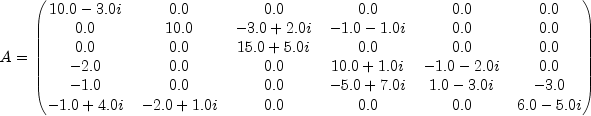JMSLTM Numerical Library 7.2.0
com.imsl.math

## Class ComplexSparseMatrix.SparseArray

• All Implemented Interfaces:
Serializable
Enclosing class:
ComplexSparseMatrix

```public static class ComplexSparseMatrix.SparseArray
extends Object
implements Serializable```
The `SparseArray` class uses public fields to hold the data for a sparse matrix in the Java Sparse Array format. This format came about as a means for storing sparse matrices in Java. In this format, a sparse matrix is represented by two arrays of arrays. One array contains the references to the nonzero value arrays for each row of the sparse matrix. The other contains the references to the associated column index arrays.

As an example, consider the following complex sparse matrix:In `SparseArray`, this matrix can be represented by the two jagged arrays, `values` and `index`, where `values` refers to the nonzero entries in A and `index` to the column indices:
```            Complex values[][] = {
{new Complex(10.0, -3.0)},
{new Complex(10.0, 0.0), new Complex(-3.0, 2.0), new Complex(-1.0,-1.0)},
{new Complex(15.0, 5.0)},
{new Complex(-2.0, 0.0), new Complex(10.0, 1.0), new Complex(-1.0, -2.0)},
{new Complex(-1.0, 0.0), new Complex(-5.0, 7.0), new Complex(1.0, -3.0),
new Complex(-3.0, 0.0)},
{new Complex(-1.0, 4.0), new Complex(-2.0, 1.0), new Complex(6.0, -5.0)}
}

int index[][] = {
{0},
{1, 2, 3},
{2},
{0, 3, 4},
{0, 3, 4, 5},
{0, 1, 5}
}
```

Serialized Form
• ### Field Summary

Fields
Modifier and Type Field and Description
`int[][]` `index`
Jagged array containing column indices.
`int` `numberOfColumns`
Number of columns in the matrix.
`long` `numberOfNonZeros`
Number of nonzeros in the matrix.
`int` `numberOfRows`
Number of rows in the matrix.
`Complex[][]` `values`
Jagged array containing sparse array values.
• ### Constructor Summary

Constructors
Constructor and Description
`ComplexSparseMatrix.SparseArray()`

• ### Methods inherited from class java.lang.Object

`clone, equals, finalize, getClass, hashCode, notify, notifyAll, toString, wait, wait, wait`
• ### Field Detail

• #### index

`public int[][] index`
Jagged array containing column indices. The length of this array equals `numberOfRows`. The length of each row equals the number of nonzeros in that row of the sparse matrix.
• #### numberOfColumns

`public int numberOfColumns`
Number of columns in the matrix.
• #### numberOfNonZeros

`public long numberOfNonZeros`
Number of nonzeros in the matrix.
• #### numberOfRows

`public int numberOfRows`
Number of rows in the matrix.
• #### values

`public Complex[][] values`
Jagged array containing sparse array values. This array must have the same shape as `index`.
• ### Constructor Detail

• #### ComplexSparseMatrix.SparseArray

`public ComplexSparseMatrix.SparseArray()`
JMSLTM Numerical Library 7.2.0